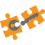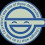## Thursday, 7 May 2009

### How to Compare two DataTables in ADO.net

Sometimes it’s very useful to compare two DataTables and return the different records to a third DataTable. I came across this when I needed to handle my own data concurrency control. Basically my logic is:

- Populate a DataTable of a DataSet and Copy the content of the DataTable to another DataTable immediately after this. (For example we populate a DataTable A and then make a copy to DataTable B.)
- When user update the DataTable A in my application, the event handler firstly populate to a DataTable C from the database.
- Comparing the DataTable C with the DataTable B, if they are same means underlying data hasn’t been changed, then proceed the update otherwise user can choose either to overwrite the data or cancel the operation.

Unfortunately you can’t do a straight comparison of two DataTables. For instance if you write something like: DataTable 1 == DataTable2 or DataTable1.GetHashCode() == DataTable2..GetHashCode() or DT1.Equals(DT2), none of this would work. Below is the code I am using to compare to DataTables, pretty self-explanatory. Hopefully you find it helpful.
`private void button1_Click(object sender, EventArgs e)   {       // Something to do with the Initialization of the FirstDataTable and SecondDataTable        DataTable dt;        dt = getDifferentRecords(FirstDataTable, SecondDataTable);        if (dt.Rows.Count == 0)            MessageBox.Show("Equal");        else           MessageBox.Show("Not Equal");   }#region Compare two DataTables and return a DataTable with DifferentRecords    public DataTable getDifferentRecords(DataTable FirstDataTable, DataTable SecondDataTable)    {        //Create Empty Table        DataTable ResultDataTable = new DataTable("ResultDataTable");        //use a Dataset to make use of a DataRelation object        using (DataSet ds = new DataSet())        {            //Add tables            ds.Tables.AddRange(new DataTable[] { FirstDataTable.Copy(), SecondDataTable.Copy() });            //Get Columns for DataRelation            DataColumn[] firstColumns = new DataColumn[ds.Tables.Columns.Count];            for (int i = 0; i < firstColumns.Length; i++)            {               firstColumns[i] = ds.Tables.Columns[i];            }            DataColumn[] secondColumns = new DataColumn[ds.Tables.Columns.Count];            for (int i = 0; i < secondColumns.Length; i++)            {                secondColumns[i] = ds.Tables.Columns[i];            }            //Create DataRelation            DataRelation r1 = new DataRelation(string.Empty, firstColumns, secondColumns, false);            ds.Relations.Add(r1);            DataRelation r2 = new DataRelation(string.Empty, secondColumns, firstColumns, false);            ds.Relations.Add(r2);            //Create columns for return table            for (int i = 0; i < FirstDataTable.Columns.Count; i++)            {                ResultDataTable.Columns.Add(FirstDataTable.Columns[i].ColumnName, FirstDataTable.Columns[i].DataType);            }            //If FirstDataTable Row not in SecondDataTable, Add to ResultDataTable.            ResultDataTable.BeginLoadData();            foreach (DataRow parentrow in ds.Tables.Rows)            {                DataRow[] childrows = parentrow.GetChildRows(r1);                if (childrows == null || childrows.Length == 0)                    ResultDataTable.LoadDataRow(parentrow.ItemArray, true);            }            //If SecondDataTable Row not in FirstDataTable, Add to ResultDataTable.            foreach (DataRow parentrow in ds.Tables.Rows)            {                DataRow[] childrows = parentrow.GetChildRows(r2);                if (childrows == null || childrows.Length == 0)                    ResultDataTable.LoadDataRow(parentrow.ItemArray, true);            }            ResultDataTable.EndLoadData();        }        return ResultDataTable;    }  #endregion`

1.Good post, many thanks.

The only issue we have found with this technique is that you get duplicate records in the resulting DataTable and no real indication of what the differences are. e.g. Inserts, Updates or Deletes. We have also attempted using the Merge function but this also errors with Constraint issues. We are trying to find a way to get the differences only and an idea of what has changed.

1.Changes that addressed this for me:

public DataTable getDifferentRecords(string resultName, DataTable FirstDataTable, DataTable SecondDataTable, bool Return1st)
{
//Create Empty Table
DataTable ResultDataTable = new DataTable(resultName);
:
:
:
if (Return1st)
foreach (DataRow parentrow in ds.Tables.Rows)
{
DataRow[] childrows = parentrow.GetChildRows(r1);
if (childrows == null || childrows.Length == 0)
}
else
//If SecondDataTable Row not in FirstDataTable, Add to ResultDataTable.
foreach (DataRow parentrow in ds.Tables.Rows)
{
DataRow[] childrows = parentrow.GetChildRows(r2);
if (childrows == null || childrows.Length == 0)
}

}

return ResultDataTable;
}

When Return1st is true: ResultDataTable contains Adds Or Updates found in SecondDataTable as compared to FirstDataTable
When Return1st is false: ResultDataTable contains Deletes Or Updates found in FirstDataTable as compared to SecondDataTable

2.Good Code but ResultDataTable is returning the empty rows as different how to solve this.

3.This comment has been removed by the author.

4.It worked perfect! Thank you!

5.This solution is flawed.
This does not account for when the two datatables return a different amount of rows.
You should implement a if check to see if FirstDataTable and SecondDataTable have the same number of rows first, before calling getDifferentRecords().

6.Just what I needed - thank you - Your method of comparison is much (much much) faster than using Enumerable.Except:

DataTable result = dtFirst.AsEnumerable().Except(dtSecond.AsEnumerable(), idColumnComparer).CopyToDataTable();

I modified your to getDifferentRecords to return either the rows of the first foreach, OR the second foreach. Commingling them didn't work for me.

Thanks again!

7.This solves DbNull issue.
if (pro.Name == column.ColumnName)
{
if(dr[column.ColumnName].GetType() == typeof(System.DBNull))
pro.SetValue(obj, string.Empty, null);
else
pro.SetValue(obj, dr[column.ColumnName], null);
}

8.This seems to have the standard issue with only working with DataTables with no more than 32 columns. Does anyone know of a solution that works with DataTables with more than 32 columns?

1.My solution (I had 40-something columns) was to combine some of them in the query (related values into comma separated values). Once in the client application, post, comparison, I would break those values up into their originally intended columns.

-- Combining Adjacent #ACR columns so there are 32 or fewer (matters for comparing inside the mCIM)
SELECT acr_number
, access_cfg
, pair_acr_number
, rdr = convert(varchar, rdr_sio) +','+ convert(varchar, rdr_number)
, strk = convert(varchar, strk_sio) +','+ convert(varchar, strk_number)
, strike = convert(varchar, strike_t_min) +','+ convert(varchar, strike_t_max) +','+ convert(varchar, strike_mode)
, door = convert(varchar, door_sio) +','+ convert(varchar, door_number)
, dc_held
, rex0 = convert(varchar, rex0_sio) +','+ convert(varchar, rex0_number)
, rex1 = convert(varchar, rex1_sio) +','+ convert(varchar, rex1_number)
, altrdr = convert(varchar, altrdr_sio) +','+ convert(varchar, altrdr_number) +','+ convert(varchar, altrdr_spec)
, cd_format
, apb = convert(varchar, apb_mode) +','+ convert(varchar, apb_in) +','+ convert(varchar, apb_to)
:
:
, strk_follow = convert(varchar, strk_follow_pulse) +','+ convert(varchar, strk_follow_delay)
, AreaID
INTO #ACR_COMBINED
from #ACR

then in my application, after the compare:

acr_number = (int) row["acr_number"];
access_cfg = (int) row["access_cfg"];
pair_acr_number = (int) row["pair_acr_number"];
rdr_sio = NthInt(row["rdr"], 0);
rdr_number = NthInt(row["rdr"], 1);
strk_sio = NthInt(row["strk"], 0);
strk_number = NthInt(row["strk"], 1);
strike_t_min = NthInt(row["strike"], 0);
strike_t_max = NthInt(row["strike"], 1);
strike_mode = NthInt(row["strike"], 2);
door_sio = NthInt(row["door"], 0);
door_number = NthInt(row["door"], 1);
dc_held = (int) row["dc_held"];
rex0_sio = NthInt(row["rex0"], 0);
rex0_number = NthInt(row["rex0"], 1);
rex1_sio = NthInt(row["rex1"], 0);
rex1_number = NthInt(row["rex1"], 1);
altrdr_sio = NthInt(row["altrdr"], 0);
altrdr_number = NthInt(row["altrdr"], 1);
altrdr_spec = NthInt(row["altrdr"], 2);
cd_format = (int) row["cd_format"];
apb_mode = NthInt(row["apb"], 0);
apb_in = NthInt(row["apb"], 1);
apb_to = NthInt(row["apb"], 2);
:
:
strk_follow_pulse = NthInt(row["strk_follow"], 0);
strk_follow_delay = NthInt(row["strk_follow"], 1);

NthInt looking like this:

private int NthInt(object obj, int n, char separator = ',')
{
return NthInt((string) obj, n, separator);
}
private int NthInt(string st, int n, char separator = ',')
{
string[] piece = st.Split(separator);
int r = 0;
try
{
r = Int32.Parse(piece[n]);
}
catch (FormatException e)
{
LogMsg(string.Format("Exception: NthInt unable to parse {0} position {1} ({2}", st, n, piece[n]), toServiceLog: true);
}
return r;
}

9.10.This comment has been removed by the author.

11.thanks you for code its working. actually i was comparing two tables rows which are firebird server database table and sqlserver database table. here from firebird server i get tables rows match with sql server database tables rows if rows are unmatched that rows should be insert in sql server database tables. i have writen for that in C# ADO.NET and i am getting error like "parent columns and child columns don't have type match" why i getting error this means firebird server database tables and sql server database tables datatype are different but column names are same so without change datatype how could be written the code. so please help me

12.This comment has been removed by the author.

13.